# Moran Processes¶

The evolutionary models discussed in the previous chapters assume an infinite population that can be divided in to infinitessimal parts. Finite populations can also be studied using a model called a Moran Process (first described in 1958).

## Moran process with neutral drift¶

Video

Consider a population of two types of fixed size $N$. This can be represented as a vector of the form: $(i, N-i)$ where $i\geq 0$ represents the number of individuals of the first type.

The term neutral drift refers to the fact that the two types reproduce at the same rate.

The Moran process is as follows:

• At a given time step: select a random individual for reproduction and a random individual for elimination
• The eliminated individual is replaced by a new individual of the same type as the individual chosen for reproduction.
• Proceed to the next time step.
• The process terminates when there is only one type of individual in the population.

Here is some simple Python code that simulates such a Process assuming an initial population of $(3, 3)$:

In :
import numpy as np
import matplotlib.pyplot as plt
%matplotlib inline

def neutral_moran(N, i=1, seed=0):
"""
Return the population counts for the Moran process with neutral drift.
"""

population = [0 for _ in range(i)] + [1 for _ in range(N - i)]
counts = [(population.count(0), population.count(1))]
np.random.seed(seed)
while len(set(population)) == 2:
reproduce_index = np.random.randint(N)
eliminate_index = np.random.randint(N)
population[eliminate_index] = population[reproduce_index]
counts.append((population.count(0), population.count(1)))
return counts

N = 6
plt.plot(neutral_moran(N=N, i=3, seed=6));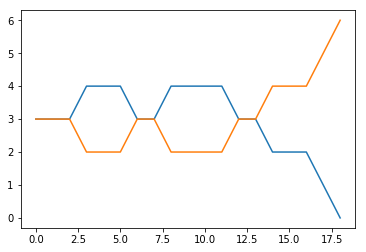For different seeds we see we obtain different results. What becomes of interest is not the path but the end result: which strategy overcomes the presence of the other?

In :
def neutral_fixation(N, i=None, repetitions=10):
"""
Repeat the neutral Moran process and calculate the fixation probability
"""
fixation_count = 0
for seed in range(repetitions):
final_counts = neutral_moran(N=N, i=i, seed=seed)
if final_counts[-1] > 0:
fixation_count += 1

return  fixation_count / repetitions


Let us take a look at probability of the first strategy taking over for different starting populations:

In :
probabilities = [neutral_fixation(N, i=i, repetitions=500) for i in range(1, N)]
plt.scatter(range(1, N), probabilities)
plt.xlabel("$i$")
plt.ylabel("$x_i$");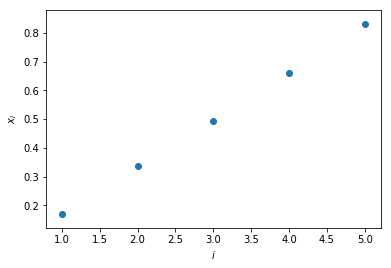We see that as the initial population starts with more of a given type, the chance that that type "takes over" (becomes fixed) grows.

This Moran Process is a specific case of a Markov Process:

• A given state of the system can be described by a single integer $0\leq i\leq N$;
• The state to state transition probabilities are given by:

\begin{aligned} p_{i, i-1}&=\frac{i(N - i)}{N^2}\\ p_{i, i+1}&=\frac{i(N - i)}{N^2}\\ p_{i, i}&=1 - p_{i, i-1} - p_{i, i+1} \end{aligned}

We also have two absorbing states (when the Moran process ends):

$$p_{00}=1\qquad p_{0i}=0\text{ for all }i>0$$

$$p_{NN}=1\qquad p_{Ni}=0\text{ for all } N>i$$

these transitions can be represented as a matrix. Here for example is the matrix for $N=6$:

In :
N = 6
p = np.zeros((N + 1, N + 1))
p[0, 0] = 1
p[N, N] = 1
for i in range(1, N):
for j in [i - 1, i + 1]:
p[i, j] = i * (N - i) / (N ** 2)
p[i, i] = 1 - sum(p[i, :])
p.round(2)

Out:
array([[ 1.  ,  0.  ,  0.  ,  0.  ,  0.  ,  0.  ,  0.  ],
[ 0.14,  0.72,  0.14,  0.  ,  0.  ,  0.  ,  0.  ],
[ 0.  ,  0.22,  0.56,  0.22,  0.  ,  0.  ,  0.  ],
[ 0.  ,  0.  ,  0.25,  0.5 ,  0.25,  0.  ,  0.  ],
[ 0.  ,  0.  ,  0.  ,  0.22,  0.56,  0.22,  0.  ],
[ 0.  ,  0.  ,  0.  ,  0.  ,  0.14,  0.72,  0.14],
[ 0.  ,  0.  ,  0.  ,  0.  ,  0.  ,  0.  ,  1.  ]])

The above corresponds to a particular type of Markov process called a Birth-Death process

## Birth death process¶

Video

A birth death process is a Markov process with the following properties:

• $p_{i,i+1}+p_{i,i-1}\leq 1$
• $p_{ii}=1-p_{i,i+1}-p_{i,i-1}$
• $p_{00}=1$ and $p_{NN}=1$

Thus we have two absorbing states: $\{0, N\}$. Let us denote by $x_i$ the probability of being in $state$ $i$ and eventually reaching state $N$.

We have the following linear system:

\begin{align} x_0&=0\\ x_i&=p_{i,i-1}x_{i-1}+p_{ii}x_i+p_{i,i+1}x_{i+1}\text{ for all }0< i< N-1\\ x_N&=1\\ \end{align}

## Theorem: Fixation probabilities for the birth death process¶

Given a birth death process as defined above, the fixation probability $x_i$ is given by:

$$x_i=\frac{1+\sum_{j=1}^{i-1}\prod_{k=1}^j\gamma_k}{1+\sum_{j=1}^{N-1}\prod_{k=1}^j\gamma_k}$$

where:

$$\gamma_k = \frac{p_{k,k-1}}{p_{k,k+1}}$$

### Proof¶

We have:

\begin{aligned} p_{i,i+1}x_{i+1} & = -p_{i,i-1}x_{i-1} + x_i(1 - p_{ii}) \\ p_{i,i+1}x_{i+1} & = p_{i,i-1}(x_{i} - x_{i-1}) + x_ip_{i,i+1} \\ x_{i+1} - x_i & = \frac{p_{i, i-1}}{p_{i, i+1}}(x_i-x_{i-1})=\gamma_i(x_i-x_{i-1}) \end{aligned}

We observe that:

\begin{aligned} x_2 - x_1 &= \gamma_1(x_1-x_{0})=\gamma_1x_1\\ x_3 - x_2 &= \gamma_2(x_2-x_1)=\gamma_2\gamma_1x_1\\ x_4 - x_3 &= \gamma_3(x_3-x_2)=\gamma_3\gamma_2\gamma_1x_1\\ &\; \vdots & \\ x_{i+1} - x_i &= \gamma_i(x_i-x_{i-1})=\prod_{k=1}^i\gamma_kx_1\\ &\; \vdots & \\ x_{N} - x_{N-1} &= \gamma_{N-1}(x_{N-1}-x_{N-2})=\prod_{k=1}^{N-1}\gamma_kx_1\\ \end{aligned}

thus we have:

$$x_i=\sum_{j=0}^{i-1}x_{j+1}-x_j=\left(1+\sum_{j=1}^{i-1}\prod_{k=1}^j\gamma_k\right)x_1$$

we complete the proof by solving the following equation to obtain $x_1$:

$$x_N=1=\left(1+\sum_{j=1}^{N-1}\prod_{k=1}^j\gamma_k\right)x_1$$

In the case of neutral drift (considered above) we have:

$$p_{i,i-1}=p_{i,i+1}$$

thus:

$$\gamma_i=1$$

so:

$$x_i=\frac{1+\sum_{j=1}^{i-1}\prod_{k=1}^j\gamma_k}{1+\sum_{j=1}^{N-1}\prod_{k=1}^j\gamma_k}=\frac{1+i-1}{1+N-1}=\frac{i}{N}$$
In :
probabilities = [neutral_fixation(N, i=i, repetitions=500) for i in range(1, N)]
plt.scatter(range(1, N), probabilities, label="Simulated")
plt.plot(range(1, N), [i / N for i in range(1, N)], label="Theoretic: $i/N$", linestyle="dashed")
plt.xlabel("$i$")
plt.ylabel("$x_i$")
plt.legend();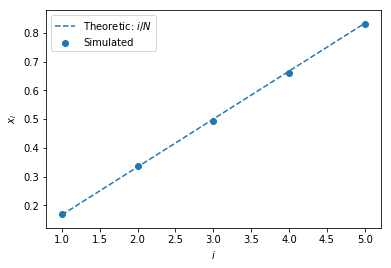## Fixation probability¶

The fixation probability in a Moran process is the probability that a give type starting with $i=1$ individuals takes over an entire population. We denote the fixation probabilities of the first/second type as $\rho_1$ and $\rho_2$ respectively and we have:

$$\rho_1=x_1$$$$\rho_2=1-x_{N-1}$$

We will now consider a Moran process on a game:

## Moran process on a game¶

Video

Consider a matrix $A\in\mathbb{R}^{m\times n}$ representing a game with two strategies.

$$A= \begin{pmatrix} a & b\\ c & d \end{pmatrix}$$

The Moran process is as follows:

• At a given time step: all individuals play all other individuals.
• Obtain their fitness as given by the game.
• Randomly select an individual proportional to their fitness as an individual to be reproduced
• Uniformly select an individual to be replaced
• Proceed to the next time step.
• The process terminates when there is only one type of individual in the population.

Assuming $i$ individuals of the first type, the fitness of both types is given respectively by:

$$f_{1i}=\frac{a(i-1)+b(N-i)}{N-1}$$$$f_{2i}=\frac{c(i)+d(N-i-1)}{N-1}$$

The transition probabilities are then given by:

$$p_{i,i+1}=\frac{if_{1i}}{if_{1i} + (N-i)f_{2i}}\frac{N-i}{N}$$$$p_{i,i-1}=\frac{(N-i)f_{2i}}{if_{1i} + (N-i)f_{2i}}\frac{i}{N}$$

which gives:

$$\gamma_i=\frac{f_{2i}}{f_{1i}}$$

thus:

$$x_i=\frac{1+\sum_{j=1}^{i-1}\prod_{k=1}^j\gamma_k}{1+\sum_{j=1}^{N-1}\prod_{k=1}^j\gamma_k}$$

Here is some code to carry out this calculation:

In :
def theoretic_fixation(N, game, i=1):
"""
Calculate x_i as given by the above formula
"""
f_ones = np.array([(game[0, 0] * (i - 1) + game[0, 1] * (N - i)) / (N - 1) for i in range(1, N)])
f_twos = np.array([(game[1, 0] * i + game[1, 1] * (N - i - 1)) / (N - 1) for i in range(1, N)])
gammas = f_twos / f_ones
return (1 + np.sum(np.cumprod(gammas[:i-1]))) / (1 + np.sum(np.cumprod(gammas)))


Here is an example of calculating $x_1$ for the following game for $N=4$:

Video

$$A = \begin{pmatrix} 4 & 1\\ 1 & 4 \end{pmatrix}$$
In :
A = np.array([[4, 1],
[1, 4]])
theoretic_fixation(N=4, i=1, game=A)

Out:
0.125

Applying the theorem gives:

\begin{aligned} f_{1i}&=\frac{4(i - 1) + 4 - i}{3} = \frac{4i-4+4-i}{3}=i\\ f_{2i}&=\frac{i + 4(3 - i)}{3} = \frac{12-3i}{3}=4-i \end{aligned}$$\gamma_i = \frac{f_{2i}}{f_{1i}}=\frac{4-i}{i}=\frac{4}{i}-1$$

Thus:

\begin{aligned} x_1 & =\frac{1 + \sum_{j=1}^{0}\prod_{k=1}^{j}\gamma_k}{1 + \sum_{j=1}^{4 - 1}\prod_{k=1}^{j}\gamma_k}\\ & =\frac{1}{1 + \sum_{j=1}^{3}\prod_{k=1}^{j}\gamma_k}\\ & =\frac{1}{1 + \gamma_1 + \gamma_1\times \gamma_2 + \gamma_1 \times \gamma_2 \times \gamma_3}\\ & =\frac{1}{1+3+3\times 1 + 3 \times 1\times \frac{1}{3}} = \frac{1}{1 + 3 + 3 + 1}=\frac{1}{8}\\ \end{aligned}

Here is some code to simulate a Moran process.

In :
def moran(N, game, i=1, seed=0):
"""
Return the population counts for
the Moran process on a 2 by 2 game
"""
population = [0 for _ in range(i)] + [1 for _ in range(N - i)]
counts = [(population.count(0), population.count(1))]

np.random.seed(seed)

while len(set(population)) == 2:

scores = []

for i, player in enumerate(population):
total = 0
for j, opponent in enumerate(population):
if i != j:
total += game[player, opponent]
scores.append(total)

total_score = sum(scores)
probabilities = [score / total_score for score in scores]
reproduce_index = np.random.choice(range(N), p=probabilities)

eliminate_index = np.random.randint(N)
population[eliminate_index] = population[reproduce_index]

counts.append((population.count(0), population.count(1)))
return counts

def fixation(N, game, i=None, repetitions=10):
"""
Repeat the Moran process and calculate the fixation probability
"""
fixation_count = 0
for seed in range(repetitions):
final_counts = moran(N=N, i=i, game=game, seed=seed)
if final_counts[-1] > 0:
fixation_count += 1
return  fixation_count / repetitions


Here is one specific simulated process for the game with initial population: $(1, 7)$ where the invader manages to become fixed.

In :
N = 8
plt.plot(moran(N=N, i=1, seed=44, game=A));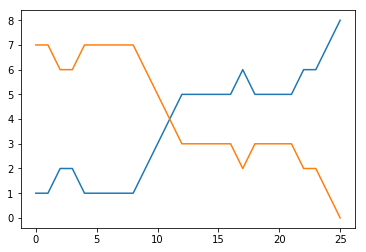Here is how the fixation probabilities vary for different initial populations:

In :
probabilities = [fixation(N, i=i, game=A, repetitions=500) for i in range(1, N)]
plt.scatter(range(1, N), probabilities, label="Simulated")
plt.plot(range(1, N), [i / N for i in range(1, N)], label="Neutral: $i/N$", linestyle="dashed")
plt.plot(range(1, N), [theoretic_fixation(N=N, i=i, game=A) for i in range(1, N)], label="Theoretic")
plt.xlabel("$i$")
plt.ylabel("$x_i$")
plt.legend();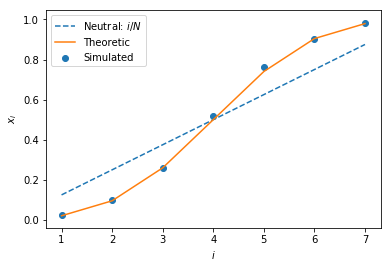Previous

Next

Source code: @drvinceknight Powered by: Python Mathjax Github pages Skeleton css